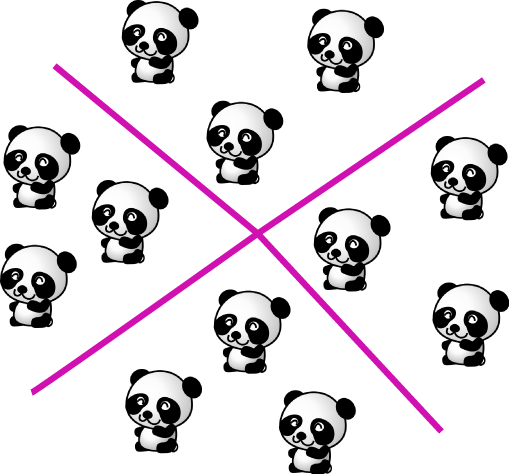# Definition of Quotient"Quotient" is the word we use to describe the answer we get when we divide a number by another number.

$\text{quotient} = \text{dividend}\div \text{divisor}$.
The dividend is the number we're dividing up, and the divisor is the number we're dividing by.

In the picture, we're dividing the $12$ pandas up into four groups. Each of the four groups contains $3$ pandas. This corresponds to the division, $12 \div 4$. The answer is $3$, so the quotient is $3$.

### Description

The aim of this dictionary is to provide definitions to common mathematical terms. Students learn a new math skill every week at school, sometimes just before they start a new skill, if they want to look at what a specific term means, this is where this dictionary will become handy and a go-to guide for a student.

### Audience

Year 1 to Year 12 students

### Learning Objectives

Learn common math terms starting with letter Q

Author: Subject Coach
You must be logged in as Student to ask a Question.